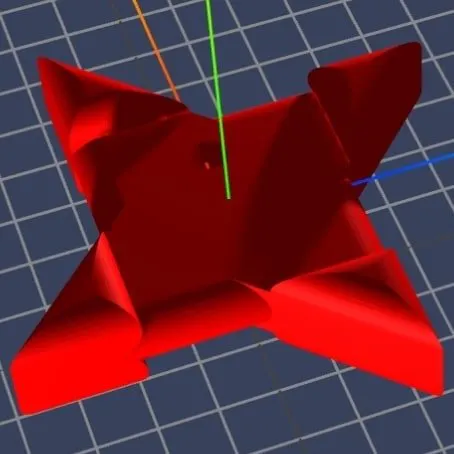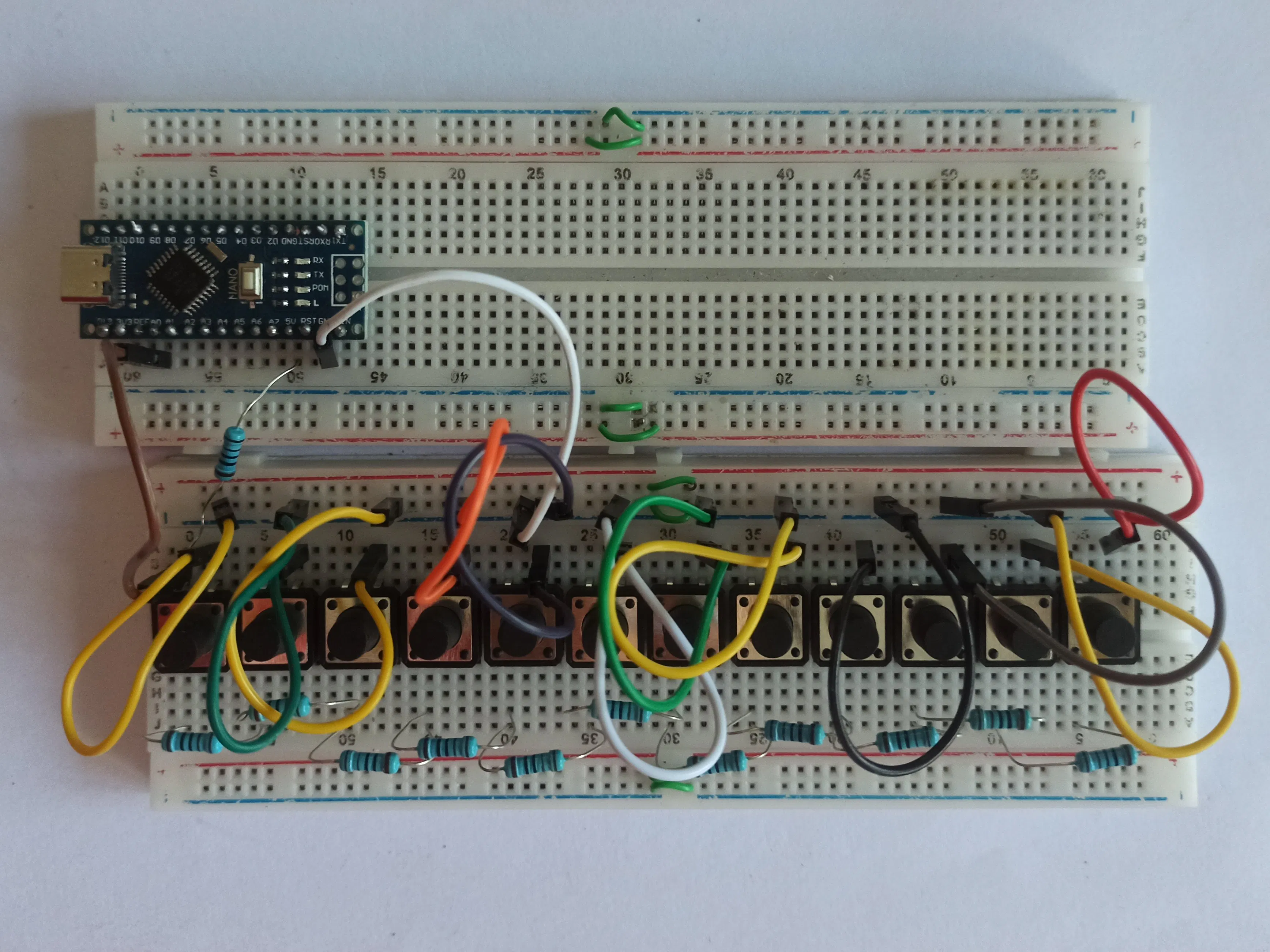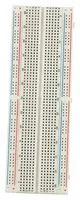# Multiple switches to single analogRead

This tutorial we will learn how to use multiple switches and output a single signal to be analogRead by an Arduino.

BeginnerFull instructions provided1 hour67## Things used in this project

### Hardware componentsArduino Nano R3
×1SparkFun Pushbutton switch 12mm
×12Resistor 1k ohm
×12

### Software apps and online servicesArduino IDE

### Hand tools and fabrication machines## Code

### Multiple switches to single analogRead

Arduino
```int pin = A0;

void setup()
{
pinMode (pin, INPUT);
Serial.begin (9600);
}

void loop()
{
Serial.println(volt);
if (volt >= 0 && volt <= 2)
{
Serial.println("1");
}
if (volt >= 510 && volt <= 514)
{
Serial.println("2");
}
if (volt >= 680 && volt <= 684)
{
Serial.println("3");
}
if (volt >= 765 && volt <= 769)
{
Serial.println("4");
}
if (volt >= 817 && volt <= 821)
{
Serial.println("5");
}
if (volt >= 851 && volt <= 855)
{
Serial.println("6");
}
if (volt >= 875 && volt <= 879)
{
Serial.println("7");
}
if (volt >= 893 && volt <= 897)
{
Serial.println("8");
}
if (volt >= 908 && volt <= 912)
{
Serial.println("9");
}
if (volt >= 919 && volt <= 923)
{
Serial.println("10");
}
if (volt >= 929 && volt <= 933)
{
Serial.println("11");
}
if (volt >= 936 && volt <= 940)
{
Serial.println("12");
}
delay (500);
}
```

## Credits

### limchengwei

17 projects • 0 followers